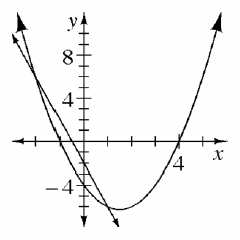### Home > AC > Chapter 10 > Lesson 10.4.3 > Problem10-149

10-149.

Solve the system of equations below by graphing. Write your solution(s) in $\left(x,y\right)$ form.

$\begin{array}{l} y=-4x-2 \\ y=x^2-3x-4 \end{array}$

If you cannot remember your graphing shortcuts, make tables and plot the points.

Where to the graphs intersect?